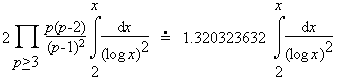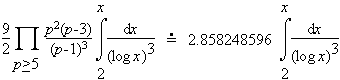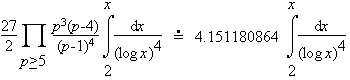# prime k-tuple conjecture

The prime k-tuple conjecture states that every admissible pattern for a prime constellation occurs infinitely often and that the number of occurrences of a prime constellation of length k is (infinitely often) greater than a constant times x/(log x)k.

Consider the cases k=2, 3, and 4. Here Hardy and Littlewood heuristically estimated the number of each pattern less than x is

 (k=2)(k=3)(k=4)Related pages (outside of this work)

References:

Guy94 (A9)
R. K. Guy, Unsolved problems in number theory, Springer-Verlag, 1994.  New York, NY, ISBN 0-387-94289-0. MR 96e:11002 [An excellent resource! Guy briefly describes many open questions, then provides numerous references. See his newer editions of this text.]
HL23
G. H. Hardy and J. E. Littlewood, "Some problems of `partitio numerorum' : III: on the expression of a number as a sum of primes," Acta Math., 44 (1923) 1-70.  Reprinted in "Collected Papers of G. H. Hardy," Vol. I, pp. 561-630, Clarendon Press, Oxford, 1966.
Ribenboim95
P. Ribenboim, The new book of prime number records, 3rd edition, Springer-Verlag, 1995.  New York, NY, pp. xxiv+541, ISBN 0-387-94457-5. MR 96k:11112 [An excellent resource for those with some college mathematics. Basically a Guinness Book of World Records for primes with much of the relevant mathematics. The extensive bibliography is seventy-five pages.]
Riesel94 (Chapter Three)
H. Riesel, Prime numbers and computer methods for factorization, Progress in Mathematics Vol, 126, Birkhäuser Boston, 1994.  Boston, MA, ISBN 0-8176-3743-5. MR 95h:11142 [An excellent reference for those who want to start to program some of these algorithms. Code is provided in Pascal. Previous edition was vol. 57, 1985.]